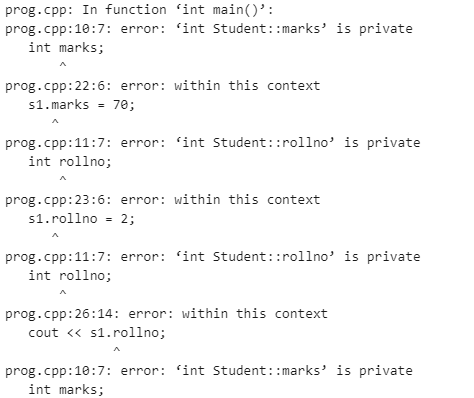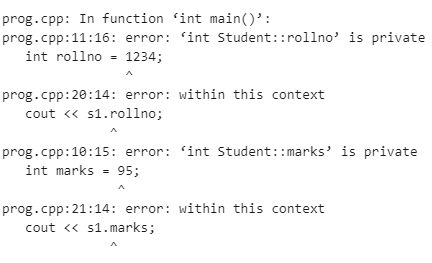# Problem of initialization in C++

• Last Updated : 18 Apr, 2022

In this article, we will discuss the problem of initialization in C++, the data members of a class have private scope by default, so they are not accessible outside the class directly. Therefore, when objects are created, the members of the object cannot be initialized directly and this problem of not being able to initialize data members is known as the problem of initialization.

Example: In the below example, a class is created with two data members.  These data members have no explicitly defined scope thus they are private by default. The private data members cannot be initialized later using the object of the class. Here, if the data members are initialized using the object of the class, then it will show an error.

## C++

 `// C++ program to demonstrate the``// above approach``#include ``using` `namespace` `std;` `// Class declaring the data members``class` `Student {``    ``int` `marks;``    ``int` `rollno;``};` `// Driver Code``int` `main()``{``    ``Student s1;` `    ``// Member variables marks and``    ``// rollno cannot be initialized``    ``// outside the class directly``    ``s1.marks = 70;``    ``s1.rollno = 2;` `    ``cout << ``"Student Roll No: "``;``    ``cout << s1.rollno;``    ``cout << ``"Student Marks: "``;``    ``cout << s1.marks;` `    ``return` `0;``}`

Output:Explanation: Now as in the above code it can be seen that private data members cannot be initialized directly outside the class.

To solve the above problem of Initialization, the concept of constructors is used. They are a special member functions that are automatically called when an object of that class is created. Also, their name is the same as the class name.

The constructors should be used to initialize member variables of the class because member variables cannot be declared or defined in a single statement. Therefore, constructors are used in initializing data members of a class when an object is created.

Below is the C++ program to illustrate the above concept:

## C++

 `// C++ program to demonstrate the``// above approach``#include ``using` `namespace` `std;` `// Class to declare the``// data members``class` `Student {``    ``int` `marks = 95;``    ``int` `rollno = 1234;` `    ``// Wrong way``};` `// Driver Code``int` `main()``{``    ``Student s1;``    ``cout << s1.rollno;``    ``cout << s1.marks;` `    ``return` `0;``}`

Output:Explanation: The above method is wrong and does not make the object an actual object because the values stored are garbage due to the private context of the class Student. The object just physically appears. To make the object behave as an actual object which can store values, constructors come into play.

Example: In the below example, a constructor is created in the class that will initialize variables with the value.

## C++

 `// C++ program to demonstrate the``// above approach``#include ``using` `namespace` `std;` `// Class student with``// student details``class` `Student {``    ``int` `marks;``    ``int` `rollno;` `    ``// Constructor of``    ``// the class``public``:``    ``Student()``    ``{``        ``marks = 95;``        ``rollno = 1234;``        ``cout << ``"Student marks: "` `<< marks << endl``            ``<< ``"Student roll no: "` `<< rollno;``    ``}``};` `// Driver Code``int` `main()``{``    ``Student s1;` `    ``// Printed Student Details``    ``return` `0;``}`
Output
```Student marks: 95
Student roll no: 1234```

My Personal Notes arrow_drop_up